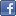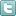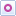EE Times-India > T&M

T&M# Power analysis: Let scopes do the math

Posted: 03 Sep 2014Print Version

Keywords:Oscilloscopes  current  voltage  power  Teledyne LeCroy

Typically, oscilloscopes are used to measure current and voltage. Through the magic of mathematics, it can also be utilised to calculate power. Unfortunately, power comes in a large number of guises: instantaneous, real, apparent, and reactive. This plethora of power terms often leads to confusion. This article explains how to use the Power Analysis software package on the Teledyne LeCroy HDO 6000 scope to eliminate the need to set up proper math operations.

Oscilloscopes, whether analogue or digital, are voltage responding instruments. Current is measured using a suitable transducer, usually a current probe or resistive shunt. The oscilloscope display is the instantaneous function of voltage or current vs. time. The product of these quantities is instantaneous power.

A basic line power measurement is shown in the figure.Figure: The elements of a power measurement (instantaneous voltage, current, and power) shown on an HDO 6000 oscilloscope equipped with the Power Analysis option. Real and apparent power are automatically computed and displayed.

The product of the instantaneous voltage (channel 1) and current (channel2) is the instantaneous power shown in the lower, line power trace. Note that the power waveform consists of a waveform at twice the frequency of the current or voltage, with a DC offset. This DC offset represents the average power being delivered to the load. The average or real power, represented by the symbol P, is measured in units of Watts. In figure 1, the real power is determined automatically by determining the mean or average value of the instantaneous power waveform. In this example, real power is displayed as the parameter rpwr and has the value 25.11 W. The product of the effective (rms) current and effective (rms) voltage is called the apparent power. Apparent power is represented by the symbol S and is measured in units of Volt-Amps (VA). In our example above, the apparent power is:

S =120.59 * 0.328 = 39.6 VA

Apparent power is automatically computed and displayed as the parameter apwr. For resistive loads, the apparent and average powers are equal.

The ratio of average to apparent power is the power factor. In the sinusoidal case, the power factor is equal to the cosine of the phase angle between the current and voltage waveforms. It is more generally computed as the ratio of real to apparent power. In our example, the power factor is also computed automatically and displayed using the parameter pf. The value of the power factor is 0.633.

Icrest is the crest factor of the current waveform. Crest factor is the ratio of the peak-to-peak value of the current to the rms value.

The reactive power, N, can be derived from the real and apparent power, using the following equation:

N = (S2—P2)1/2

The units of reactive power are Volt Amperes Reactive or VAR. Most users have an interest in real power and power factor, so reactive power is not calculated automatically.

 Related Articles Editor's Choice
Comment on "Power analysis: Let scopes do the ma..."

Top Ranked Articles

WebinarsVisit Asia Webinars to learn about the latest in technology and get practical design tips.

Search EE Times India
Services

Go to topConnect on FacebookFollow us on TwitterFollow us on Orkut
﻿